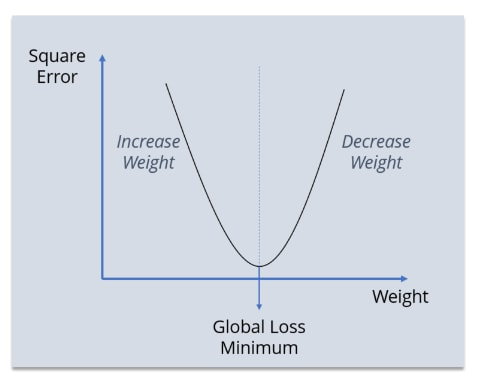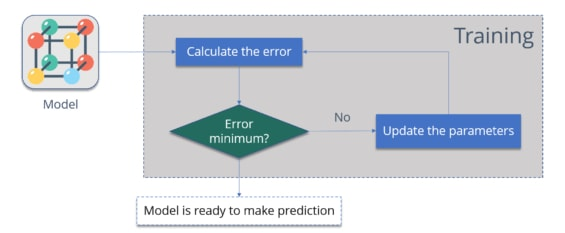# What is BackPropagation?

May 31st, 2019

## What is BackPropagation?

Backpropagation is an algorithm used for training neural networks. When the word algorithm is used, it represents a set of mathematical- science formula mechanism that will help the system to understand better about the data, variables fed and the desired output.

### Definition

Backpropagation is a kind of method to train the neural network to learn itself and find the desired output set by the user.
Neural networks are layers of networks arranged like to represent the human brain with weights (connecting one input to another). This arrangement of layers of inputs is called input neurons, they are followed by their successor called output neurons. The connecting points of these neurons are called weights. The weights transmit the input data from one neuron to another via the layers.
The mechanism of transmission of data from one input neuron to another and to the next one is called learning or training of the neural network.
This learning will be either with supervision or unsupervised. Supervised learning, has desired and defined outputs. Unsupervised is for grouping the data and representing them based on their similarities shared.
With all the important basic foundations explained, now backpropagation is the kind of supervised learning method. It is called an algorithm because it measures, finds the formula to relate the input variables.

#### Little bit History

• In the year 1961, J. Kelly, Henry Arthur, and E. Bryson wrote the basics concept of continuous backpropagation
• In the year 1969, Bryson and Ho derived a multi-stage dynamic system for optimization methodologies.
• Later in 1974, the facts for applying the above methodology principle in any kind of neural system
• In the year 1982, Hopfield established his unique neural network with his own idea.
• Around1986, efforts put by the following men in the back propagation was recognized globally.
• David E. Rumelhart
• Ronald J. Williams,
• Geoffrey E. Hinton
• Finally in the year 1993, Wan won the first international pattern recognition model in a contest using the backpropagation method.

### Logic operation of Backpropagation Algorithm

Backpropagation- when written separately, it is Back-propagation,

• Back – send backward
• Propagate – ability or the action of to transmit something

The inputs are sent backward in the network. Why should you send it backward? The answer needs to be explained in an elaborate manner. The neural network is like just born-babies who literally knew nothing about the world. When you want the AI to be powered with 100% efficiency, you need the neural network to be trained so strongly that it can interpret any kind of data given to it. In other words, it is an untouched or unwrapped device that left as it becomes useless.
When you train the newly created network, it is natural for producing errors and make mistakes. Only from the mistakes, the network learns to find the defined output. And backpropagation falls under supervised learning; under the supervision of a teacher who is training the network to deliver the correct output.

#### Example of Backpropagation Algorithm

When a shooter is striking an object that is thrown at the air, you want the system to calculate the velocity of his bullet striking the object. At first, you have given a set of input that identifies only the distance between the target and the shooter. The answer is wrong since the direction is important because velocity has direction. So now the error is measured, it is propagated backward and now the weights will take the direction of the bullet and also the angle it is projected, which might alter the force and can reduce or increase the velocity.
In the above model, the first output produced is not the desired output, so now the network measures the error value between the actual output and desired output. With this, the algorithm helps to increase or decrease the value to find the next set of output. If that matches the desired output, the network will not propagate anymore backward. In case, the actual output value results in higher error value while compared with the desired output, then the values of the weights have to be decreased. This is measured with the term called “Gradient Descent”.An optimization factor that will find the minimum value needs to be used to get any desired output. In machine learning, gradient descent will try to update the parameters (proportional to negative function at that current point).
The ultimate aim of the factor is to achieve global loss minimum. In simple words, get the desired output with a minimum loss value (reduce the number of error repetitions).

### Algorithm Functioning Prototype

The real formula behind the backpropagation algorithm is quite tedious, but let us try to understand them with simple functions.
The following are the functions

• The f(x) input to be given is 0 and the output has to be 0
• The f(x1) input to be given is 1 and the output has to be 2
• The f(x2) input to be given is 2 and the output has to be 4
• The f(x3) input to be given is 3 and the output has to be 6
• The f(x4) input to be given is 4 and the output has to be 8

So the output is the doubled value of the input fed.

#### Case- 1

Now, when you feed the input 1 to the network, the neurons transmit them through the weights, and it passes through the layers but the output is 3. This output is not our desired output. It is labeled as Model output when W=3. (W is weighted)
Error value = Difference between the desired output and actual output obtained
Absolute Error value = 3-2 = 1
The square error value is 1
The network now propagates the data backward and alters the weight value, either increases the weight value or decreases it. First, let us try by increasing the weight value.

#### Case – 2

Now we will increase the W=4
Now, when you feed the input 1 to the network, the neurons transmit them through the weights, and it passes through the layers but the output is 4.
Absolute Error value = 4-2 = 2
The square error value is 4
As you can see the error value has increased than the first time, so it means the network now learns that the desired output cannot be achieved by increasing the weight value again. So now it sends backward the transmission and tries to decrease the weight value.

#### Case – 3

Now we will decrease the W=2
Now, when you feed the input 1 to the network, the neurons transmit them through the weights, and it passes through the layers and the output is 2.
Absolute Error value = 2-2 = 0
The square error value is 0
BINGO! The desired output matches with the actual output and the error value are zero. Now the network has learned to understand that the f(x) function needs to be doubled the value given to the x.

### Summarizing the Algorithm BehaviorStep 1: Get the input dataset – feeding the input values to the neural network.
Step 2: Network predicts an output by applying a random parameter value to its weights
Step 3: Calculating the error set value
Step 4: Update the parameters – Increase or decrease the value to a range to check if the error value is being minimized or not
Step 5: Check for the error value is minimized or increased
Step 6: Update the parameter value again
Step 7: Iterate the process until the error value attains the zero.

### Types of Networks in Backpropagation

#### Static Backpropagation

A model where you have a static set of inputs and need them to map to a static set of output. Your input will not change or exhibit a dynamic nature. Ex: Characters recognition

#### Recurrent Backpropagation

In this model, a fixed value is achieved by propagating it forward and then after that error value is calculated by propagating it backward.
The overall difference is that mapping is quick and fast (rapid) in the static model and non-static in the recurrent model since the inputs will have dynamic functions.

• Useful in the high error-prone projects like facial recognition, character recognition.
• The performance and the speed of the neural network are increased since the model has the ability to remove the weights which have minimal value on the network.
• Compared to other algorithm models, this network is the simplest way to train a neural network fastly.

• Highly sensitive. The algorithm model is sensitive to noisy data.
• Dependent on the input dataset. This limits the functionality and only establishes a relationship when you have a known input dataset.
• Cannot do a mini-batch based approach. Will require a matrix method format.

#### Conclusion

Neural networks, AI and machine learning are taking over the world. They say, “Humans are hooked but machines are learning”. You will feel the phrase is real every inch when you just get a small peek into the AI technology and the wonders that are being created with it. It may not be surprising if you know that 50% of the business and IT firms are into machine learning to automate many of their processes. Let us wait for the advanced miracles like drones delivering courier packages and automatic public vehicles to be found very frequently in every neighborhood.

## Related Blogs:

• ##### What is Artificial Neural Network?
• What is Machine Learning?
Social Share: